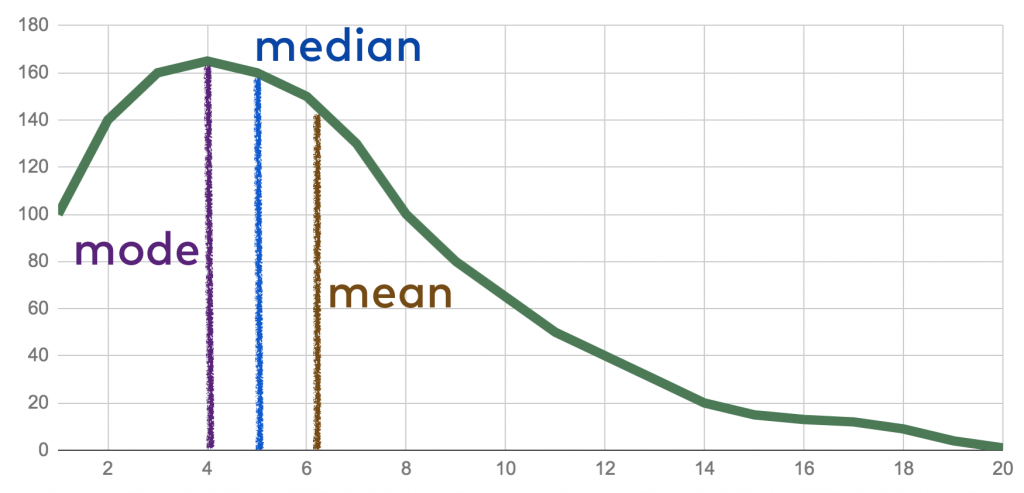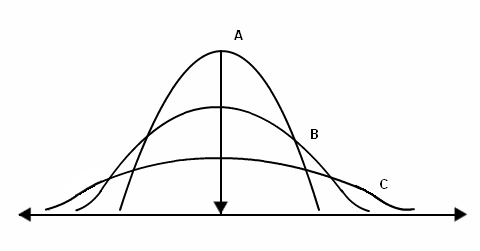Statistics for SSC-CGL 2020 - Study24x7# Welcome Back

or

By Registering, you agree to our and of use.

# Statistics for SSC-CGL 2020

Published on 15 February 2020The aspirants who are preparing for SSC CGL 2020 must understand what SSC CGL is.

The SSC CGL 2020 age limit and syllabus for SSC CGL 2020 has been already mentioned on their website https://ssc.nic.in/

Collection Classification and Presentation of Statistical Data for SSC-CGL 2020

In statistics, the raw data is collected and classified on the basis of different categorizations to simplify the further process.

Collection of Statistical Data: Data can be collected in three ways:

Questionnaire method

Interview method

Registration method

Classification of Statistical Data:

Classification of statistical data includes the process of aligning data into a particular order on the basis of some common characteristic from the available raw data.

In statistics, data can be classified in the following ways:

1. Qualitative Classification

2. Quantitative Classification

3. Chronological Classification

4. Geographical Classification

5. Conditional Classification

Representation of data:

Textural Method: This method is used on data for synthesis and generalization purposes.

Tabular Method: It represents the data in row and column-wise and provides a way to compare it easily.

Semi tabular Method: It includes both text and tables in its representation.

Graphical Representation: It represents the data in the form of different charts or graphs like a pie chart, bar graph, etc.Measures of Central Tendency for SSC CGL 2020

It is a single representation of a mass set of data.

There are three main measures of central tendency.

These measures are mean, median, mode.

Mean: It gives a single value for a mass set of data by adding all observations present in the dataset and divide it by the total number of values in the dataset. Here scope for estimation is not present.

Mean= addition of all observations/ total number of observations

Median: It gives the middle value of an arranged dataset where an arrangement of the values is in ascending order.

When a dataset has an odd number of observations:

Median = Middle value of dataset

When dataset has even number of observations:

Median= Mean of two middle values

It divides the distribution of the dataset in half.

Mode: It is the value defined on the basis of the occurrence of any value in the dataset. It gives the value of the most observed data element in the dataset.

It can be determined for quantitative and categorical both types of data.

It cannot represent the centre of distribution for a dataset.Measures of Dispersion for CGL 2020

It represents the scattering of data. It shows the deviation of data from central distribution.

It is used to compare different data sets and series.

Different measures of dispersion are as follows:

Range

Quartile Deviation

Interquartile range

Mean Deviation

Standard Deviation

Lorenz Curve

There are two categories of measure of dispersion.

These are as follows:

Absolute measure of dispersion: It is measured in terms of actual values of datasets.

For example,

Range= Dmax- Dmin

Where D is the distance between two values.

Relative measure of dispersion: It is used for comparing the two or more data set of different units. It is represented in the form of the ratio of averages.Hope you find our article for your SSC-CGL 2020 Preparation !

Write a comment...